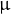# 4.6 Working with physical units

Pyxplot, and all its mathematical functions, have native support for numbers to have physical units. This means, for example, that multiplying two lengths yields an area, and taking passing a selection of lengths to the max(...) function returns the longest of the lengths supplied.

This makes it a powerful desktop tool for converting measurements between different systems of units – for example, between imperial and metric units – or for doing physical calculations.

The special function unit() is used to specify the physical unit associated with a quantity. For example, the expression

```print 2*unit(s)
```

takes the number 2 and multiplies it by the unit s, which is the SI abbreviation for seconds. Technically, the function unit(s) returns a numeric object equal to one second.

The resulting quantity above, which has dimensions of time, could then, for example, be divided by the unit hr to find the dimensionless number of hours in two seconds:

```print 2*unit(s)/unit(hr)
```

Compound units such as miles per hour, which is defined in terms of two other units, can be used as in

```print 2*unit(miles/hour)
```

In many cases, commonly-used units such units have their own explicit abbreviations, in this case mph:

```print 2*unit(mph)
```

As these examples demonstrate, the unit() function can be passed a string of units either multiplied together with the * operator, or divided using the / operator. Units may be raised to powers with the ** operator1, as in the example:

pyxplot> a = 2*unit(m**2)
pyxplot> print "An area of %f square feet"%(a/unit(ft**2))
An area of 21.527821 square feet

As the examples above have demonstrated, units may be referred to by either their abbreviated or full names, and each of these may be used in either their singular or plural forms. For example, s, second, and seconds are all valid and equivalent. A complete list of all of the units which Pyxplot recognises by default, together with all of their recognised names, can be found in Appendix 15.

SI units may be preceded with SI prefixes, as in the examples2:

```a = 2*unit(um)
a = 2*unit(micrometers)
```

When quantities with physical units are substituted into algebraic expressions, Pyxplot automatically checks that the expression is dimensionally correct before evaluating it. For example, the following expression is not dimensionally correct and would return an error because the first term in the sum has dimensions of velocity, whereas the second term is a length:a = 2*unit(m)b = 4*unit(s)print a/b + a

Pyxplot continues to throw an error in this case, even when explicit numerical errors are turned off with the set numeric errors quiet command, since it is deemed a serious error: the above expression would never be correct for any values of a and b given their dimensions.

A large number of units are pre-defined in Pyxplot by default, a complete list of which can be found in Appendix 15. However, the need may occasionally arise to define new units. It is not possible to do this from an interactive Pyxplot terminal, but it is possible to do so from a configuration script which Pyxplot runs upon start-up. Such configuration scripts will be discussed in Chapter 19. New units may either be derived from existing SI units, alternative measures of existing quantities, or entirely new base units such as numbers of CPU cycles or man-hours of labour.

Footnotes

1. Thecharacter may be used as an alias for the ** operator, though this notation is arguably confusing, since the same character is used for the binary exclusive or operator in Pyxplot’s normal arithmetic.
2. As the first of these examples demonstrates, the letter u is used as a Roman-alphabet substitute for the Greek letter.